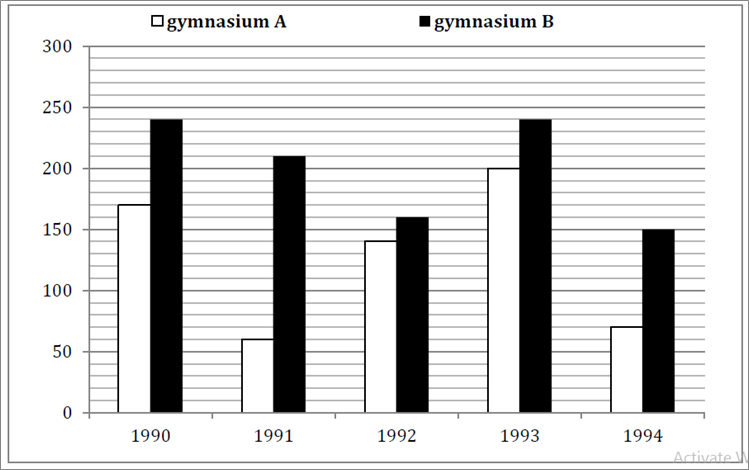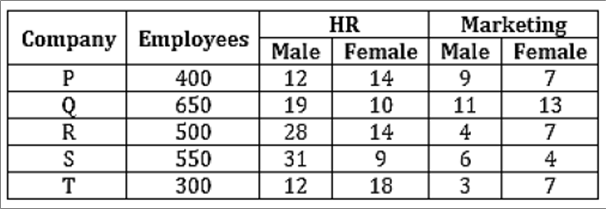# SBI Clerk Numerical Ability Online Test Questions Test 2

1.
Directions(Q.1 to Q.5 ):

In the Bar-chart, total members enrolled in different years from 1990 to 1994 in two gymnasium A and B. Based on this Bar chart solve the following questions-If in the year 1995 there is 30% increase in total number of members enrolled then in 1994 by both gymnasium, then find the total no. of members enrolled in 1995?

a

282

b

296

c

292

d

286

e

none of these

2.

The ratio between total members of both gymnasium in 1991 to total members in 1994 of both gymnasium is-

a

22:27

b

21:11

c

11:21

d

25:13

e

27:22

3.

The number of members of gymnasium A in 1991 is what % of the no. of members of gymnasium B in 1994.

a

60%

b

55%

c

58%

d

62%

e

none of these

4.

The total number of members enrolled in gymnasium A from 1991 to 1994 together is what percent more than the total number of members enrolled in gymnasium B in 1993 and 1994 together?(Rounded off to 2 decimal places)

a

10.51%

b

20.51%

c

15.51%

d

17.51%

e

none of these

5.

Total member enrolled in gymnasium B in 1993 and 1994 together is what % more than members enrolled in gymnasium A in 1990 and 1994 together?

a

60%

b

65%

c

62.5%

d

61.5%

e

none of these

6.
Directions(Q.6 to Q.10 ):

What should come in place of question mark (?) in the following number series?

4,3,4,7,15, ?

a

38.5

b

40

c

45

d

37.5

e

none of these

7.

7,5,7,17,63, ?

a

321

b

309

c

305

d

301

e

none of these

8.

11,14,19,28,43, ?

a

60

b

63

c

66

d

70

e

none of these

9.

2 60 10 120 30 ?

a

222

b

216

c

208

d

230

e

None of these

10.

23 50 108 232 492 ?

a

1028

b

1024

c

1020

d

1032

e

None of these

11.
Directions(Q.11 to Q.15 ):

There are five companies and we have given the no. of employee working in different companies. In the table we have also given the percentage of male and female employees of HR and Marketing department.If 60% of the employees of company T in HR department have MBA degree and 40% of the employees of the same company in the Marketing dept. have MBA degree, then how many employees have MBA degree in company T in both dept. together.

a

98

b

108

c

106

d

92

e

66

12.

Find the ratio of female employees of company Q in HR dept. to male employees of company R in Marketing dept. ?

a

4:13

b

5:22

c

22:5

d

13:4

e

none of these

13.

Total number of HR employees of company P is what % more than the total no. of marketing employee in company T?

a

236.76%

b

226.67%

c

276.76%

d

246.67%

e

none of these

14.

The ratio of male employees in HR dept. of company P and R together to female employees of Marketing department in company S and T together?

a

187:27

b

43:188

c

188:43

d

27:187

e

none of these

15.

Difference between female employees of HR dept. in all companies together (excluding company S) and the female employees of Marketing dept. in all companies together (excluding company Q)?

a

139

b

129

c

135

d

141

e

none of these

16.
Directions(Q.16 to Q.25 ):

A mixture contains wine and water in the ratio 3 : 2 and another mixture contains them in the ratio 4 : 5. How many litres of the latter must be mixed with 3 litres of the former so that the resultant mixture may contain equal quantities of wine and water ?

a

$1frac{2}{3}{ m{litre}}$

b

$5frac{2}{5}$ ${ m{litre}}$

c

$4frac{1}{2}{ m{litre}}$

d

$3frac{3}{4}$ litre

e

none of these

17.

A trader sells two bullocks for Rs. 8,400 each, neither losing nor gaining in total. If he sold one of the bullocks at a gain of 20%, the other is sold at a loss of

a

20%

b

18$frac{2}{9}$%

c

14$frac{2}{7}$%

d

21%

e

None of these

18.

minutes and 3 hours 20 minutes to reach Y and X respectively after they meet. If train A is moving at 45 km/hr., then the speed of the train B is

a

60 km/hr

b

64.8 km/hr

c

54 km/hr

d

37.5 km/hr

e

None of these

19.

Out of his total income, Mr. Kapoor spends 20% on house rent and 70% of the remaining on house hold expenses. If he saves Rs 1,800 what is his total income (in rupees)?

a

Rs 7,800

b

Rs 7,000

c

Rs 8,000

d

Rs 7,500

e

None of these

20.

A can do a piece of work in 8 days which B can destroy in 3 days. A has worked for 6 days, during the last 2 days of which B has been destroying. How many days must A now work alone to complete the work?

a

7 days

b

7$frac{1}{3}$ days

c

7 $frac{2}{3}$ days

d

8 days

e

None of these

21.
Directions(Q.21 to Q.25 ):

What approximate value should come in place of the question mark (?) in the following questions? (You are not expected to calculate the exact value.)

57% of 394-2.5% of 996=?

a

215

b

175

c

200

d

180

e

227

22.

96.996×9.869+0.96=?

a

860

b

870

c

1080

d

965

e

1100

23.

$frac{3}{5} imes frac{{1125}}{{1228}} imes 7 = ?$

a

7

b

12

c

9

d

4

e

15

24.

$left( {sqrt {329} imes 25} ight) div 30 = ?$

a

12

b

15

c

24

d

21

e

9

25.

(638+9709−216)÷26=?

a

275

b

345

c

440

d

300

e

390

26.
Directions(Q.31 to Q.40 ):

A and B are partners in a business. They invest in the ratio 5 : 6, at the end of 8 months A withdraws. If they receive profits in the ratio of 5 : 9, find how long B’s investment was used?

a

12 months

b

10 months

c

15 months

d

14 months

e

18 months

27.

There are 3 red balls, 4 blue balls and 5 white balls. 2 balls are chosen randomly. Find probability that 1 is red and the other is white.

a

5/22

b

5/23

c

7/22

d

4/9

e

None of these

28.

According to a new plan rolled out by HISP Bank, the rate of simple interest on a sum of money is 8% p.a. for the first two years, 10% p.a. for the next three years and 6% p.a. for the period beyond the first five years. Simple interest accrued on a sum for a period of eight years is Rs. 12,800. Find the sum.

a

Rs. 24, 000

b

Rs. 16, 000

c

Rs. 15, 000

d

Rs. 13,500

e

None of these

29.

Three Science classes A, B and C take a Life Science test. The average score of students of class A is 83. The average score of students class B is 76. The average score of class C is 85. The average score of class A and B is 79 and average score of class B and C is 81. Then the average score, of classes A, B and C is

a

80

b

80.5

c

81

d

81.5

e

None of these

30.

A hemispherical bowl of internal diameter 54 cm contains a liquid. The liquid is to be filled in cylindrical bottles of radius 3 cm and height 9 cm. How many bottles are required to empty the bowl?

a

221

b

343

c

81

d

243

e

162

Rate This:
NaN / 5 - 1 votes

### SBI Clerk Numerical Ability Online Test Questions Test 2

» Your comments will be displayed only after manual approval.